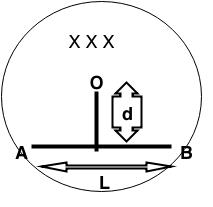# EMI 3Consider a cylindrical region containing a magnetic field.

The field is directed into the plane of the screen and is changing at a constant rate K (K>0)

A metallic rod (AB) is placed as shown, at a distance d from the center. The length of the rod is L.

(O is not connected to the rod, just ignore the vertical line from O to the rod)

The rod is completely contained within the circle.

Find the potential difference between points B and A when the net electric field in the rod becomes zero.

i.e find, ${ V }_{ B }-{ V }_{ A }$

DETAILS

• d = 2 m

• L = 2m

• K = 1

• If your answer is negative (say, negative 3) write it as -3

HINT

Scalar potential is defined for one type of electric field only.

×